Courses

# Power System 8

## 10 Questions MCQ Test Mock Test Series for Electrical Engineering (EE) GATE 2020 | Power System 8

Description
This mock test of Power System 8 for Electrical Engineering (EE) helps you for every Electrical Engineering (EE) entrance exam. This contains 10 Multiple Choice Questions for Electrical Engineering (EE) Power System 8 (mcq) to study with solutions a complete question bank. The solved questions answers in this Power System 8 quiz give you a good mix of easy questions and tough questions. Electrical Engineering (EE) students definitely take this Power System 8 exercise for a better result in the exam. You can find other Power System 8 extra questions, long questions & short questions for Electrical Engineering (EE) on EduRev as well by searching above.
QUESTION: 1

### The steady state stability limits for round rotor and salient pole 3 – phase synchronous generator are attained at the value of power angle δ

Solution:

Maximum steady state stability Limit 8 is attained at a power angle of 90° for round rotor and less than 90° for salient pole 3 - ϕ synchronous generator i.e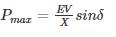*Answer can only contain numeric values
QUESTION: 2

### The inertial constant of 500 MVA, 13 kV generator is 8 MJ/MVA. The kinetic energy stored in the rotor at the synchronous speed is – (in MJ)

Solution:

Inertia constant(H) =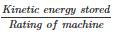⇒ kinetic energy stored = 500 MVA × 8 MJ/MVA

= 4000 MJ.

QUESTION: 3

### An alternator is connected to an infinite bus as shown in the figure. It delivers 1.0 p.u. current at 0.707 p.f lagging at V = 1.0 p.u. The reactance Xd of the alternator is 1.4 p.u. keeping the active power fixed, if the excitation reduced, the critical excitation corresponding to operation at stability limit is ______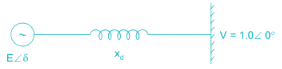Solution:

Active power output (Pe) = VI cosθ

= 0.707 p.u.

steady state power limit = EV/Xd
by keeping active power fixed if the excitation is reduced, then the critical excitation corresponding to operation at stability limit be E1.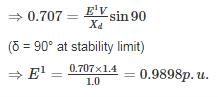*Answer can only contain numeric values
QUESTION: 4

A 50 Hz. 4 pole turbo generator rated 200 MVA, 13 kV has an inertia constant of 6 MJ/MVA. If mechanical input is suddenly raised to 100 MW for an electrical load of 80 MW, then its rotor accelerations – (in elec-deg/sec2)

Solution:

Given that,

S = 200 MVA

H = 6 MJ/MVA

F = 50 Hz

Pa = Ps – Pe = 100 – 80 = 20 Mw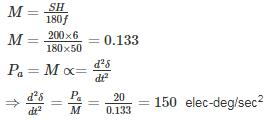QUESTION: 5

A synchronous generator is feeding 250 MW to a large 50 Hz network over a double circuit transmission line. The maximum steady state power that can be transmitted over the line with both circuits in operation is 500 MW and is 350 MW with any one of the circuits. A solid three phase fault occurring at the network end of one of the lines causes it to trip. Calculate the critical clearing angle in which the circuit breakers must trip so that synchronism is not lost.

Solution: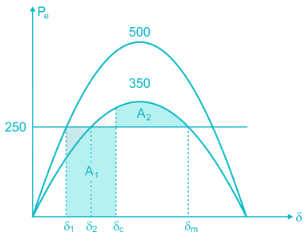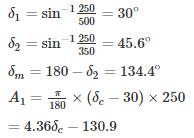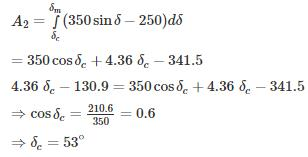*Answer can only contain numeric values
QUESTION: 6

A 50 Hz synchronous generator is connected to an infinite bus through a line. The pu. reactances of the generator and the lines are j 0.4 pu and j 0.2 pu respectively. The generator no-load voltage is 1.2 pu and that of the infinite bus is 1.0 pu. The inertia constant of the generator is 4 MW-Sec/MVA. Determine the frequency (in Hz) of natural oscillations if the generator is loaded to 70% of its maximum power transfer capacity and small perturbation is given

Solution: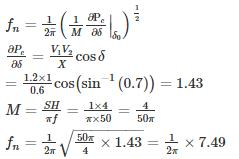fn = 1.19 Hz.

QUESTION: 7

A generator is delivering 1.0 pu power shown in the figure. A three-phase fault occurs at point F. The critical clearing angle for clearing the fault with simultaneous opening of breaker 1 and 2 is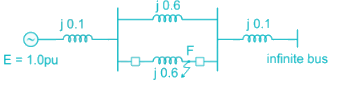Solution:

Before fault, x1eq = j0.1 + (j0.6||j0.6) + j0.1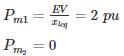= j0.1 + j0.3 + j0.1 = j0.5
After fault, x3eq = j0.8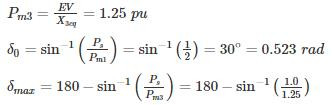= 126.86° = 2.214 rad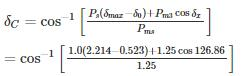⇒ δc = 41.15°

*Answer can only contain numeric values
QUESTION: 8

A 100 MVA generator operates on full load of 50 Hz frequency. The load is suddenly reduced to 60 MW. The system value begins to close only after 0.5 sec. and if the value of the inertia constant (H) is 4 MJ/MVA, then the frequency at 0.5 sec is – (in Hz)

Solution: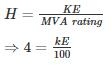⇒ KE = 400 MJ

KE stored in 0.5 sec = 60 × 0.5 = 30 MJ

KE is directly proportional to square of the frequency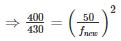⇒ f = 51.84 Hz

*Answer can only contain numeric values
QUESTION: 9

In a power system has pre-fault voltage 1 PU and ZBUS given as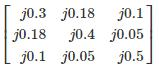If 3ϕ fault occur at bus 3 find the post-fault voltage at bus 2 is ______ (in PU)

Solution: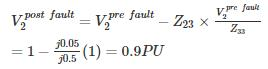QUESTION: 10

In the system shown in the figure, a static three-phase capacitor of reactance 1 pu per phase is connected through a switch at motor bus bar. The internal voltage and reactances are specified. The steady state power limits when the switch is open and closed respectively are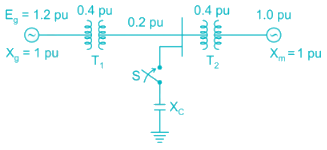Solution:

When switch is open, the reactance diagram is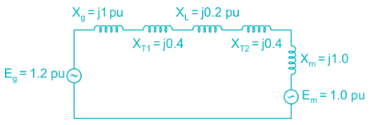xeq = j1 + j0.4 + j0.2 + j1 = j3.0 pu

Steady state power limit =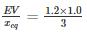= 0.4 pu

When switch is closed, the reactance diagram is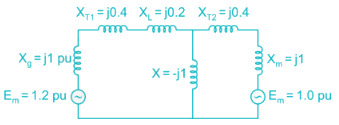By converting star in to delta,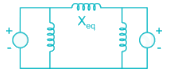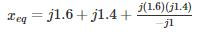= 0.76 pu
Steady state power limit =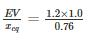= 1.58 pu